# Important Questions For Class 12 - Physics - Chapter 3 - Current Electricity

When an electric field is present, an electric charge is subject to a force, which causes it to move if it is free to do so. This movement of charge is known as a current. There are some instances in nature where free-charged particles can be found, such as in the ionosphere. However, in most matters, such as atoms and molecules, the negatively charged electrons and positively charged nuclei are bound together and unable to move freely. A large amount of matter, such as a gram of water, is made up of numerous molecules that are so densely packed that the electrons are no longer connected to specific nuclei. Some materials, like insulators, have electrons that are bound and will not accelerate when an electric field is applied, but other materials, like conductors, have electrons that are largely free to move within the bulk material. As a result, when an electric field is applied, these materials develop electric currents.

When there is no electric field present, the electrons will still be in motion due to random collisions with fixed ions caused by thermal energy. These collisions do not affect the speed of the electrons, but they can change the direction of their movement. As there is no preference for the direction of the velocities of electrons at a particular time, the number of electrons moving in any given direction will be equal to the number moving in the opposite direction. As a result, on average, there is no net movement of charge, and no electric current will be observed.

In metallic conductors, the charges that are able to move and contribute to electrical current are the electrons. These electrons come from the outermost (valence) shells of atoms in the metal and are able to move freely within the metal but are not able to leave it. They move randomly, colliding with each other and with the metal ions, and in the presence of an external electric field, they will drift in the opposite direction of the field. The positive charges in metal are made up of the nuclei of atoms, which remain in fixed positions, and the negatively charged electrons in the inner shells of atoms, which also remain fixed. In electrolytic conductors, the charge carriers are positive and negative ions, and their movement is influenced not only by an external electric field but also by chemical forces within the material.

Important Questions for Class 12 Physics Chapter 3 – Current Electricity are provided here. Students must go through these questions and solve them to prepare for their Physics papers. They can also refer to these questions for quick revision. These questions are more likely to be asked in the exam; hence, students must practise them thoroughly.

## Very Short Answer Type Questions

1. Which of the options given is non-ohmic resistance?

(a) Copper wire

(b) Lamp filament

(c) Diode

(d) Carbon resistor.

Answer: (C) Diode has non-ohmic resistance in nature.

Explanation: A diode delivers a constant voltage drop even if there is a change in the current, so it does not follow Ohm’s law.

2. What is the unit of conductance?

(a) Siemen

(b) Dyne

(c) Volts

(d) Ohm

Explanation: The unit used to measure conductance is called siemen.

3. Current density is a __________.

(a) vector quantity

(b) dimensionless quantity

(c) scalar quantity

(e) None of the choices is suitable

Explanation: Current density is a vector that describes the flow of electric current per unit area. It is a product of charge density and velocity. Here charge density is a scalar quantity, and velocity is a vector quantity, which makes current density a vector quantity.

4. _________ is the phenomenon in which the resistance of certain materials drops to zero when cooled below a specific temperature.

(a) Conductivity

(b) Superconductivity

(c) Partial conductivity

(d) Insulation

Explanation: Superconductivity is the phenomenon in which the resistance of certain materials drops to zero when cooled below a specific temperature.

5. Is the following statement true or false: The overall resistance in a series circuit is higher than the greatest resistance present in the circuit?

(a) True (b) False

Explanation: In a series circuit, the overall resistance is greater than the highest individual resistance present in the circuit.

6. What is the resistance that the electrolyte in a cell provides to the flow of current through it is referred to as?

(a) Internal resistance

(b) Non-resistance

(c) External resistance

(d) None of the choices is suitable

Explanation: The internal resistance of a cell refers to the resistance provided by the electrolyte to the flow of current through it.

1. Is the motion of a charge across junction momentum conserving? Why or why not?

Solution:

If the motion of a charge is occurring across a junction, the momentum of the charge is not at all conserved. This is because when the charge (electron) approaches the junction, apart from the uniform electric field that it typically encounters (which maintains the drift velocity fixed), there will be an accumulation of charges on the wires’ at the junction. This also generates an electric field, enabling the change in the direction of the charge’s momentum.

2. The relaxation time ‘t’ is nearly independent of the applied E field, whereas it changes significantly with temperature T. First fact is (in part) responsible for Ohm’s law, whereas the second fact leads to the variation of p (rho) with temperature. Elaborate why?

Solution:

When the drift velocity rises, the relaxation time ‘t’ (the average time between consecutive collisions) falls, which increases‘p (rho)’ by the relation given below:

$$\begin{array}{l}\rho = \frac{ m }{ n e^2 \tau }\end{array}$$

The drift velocity vd varies in the range of one mm on the rising electric field. On the other hand, the drift velocity rises of the order 102ms-1 when the count of free electrons rises with increasing temperature.

Therefore, due to the rise in vd the relation time considerably decreases in conductors.

3. What are the advantages of the null-point method in a Wheatstone bridge? What additional measurements would be required to calculate Runknown by any other method?

Solution:

In the case of a Wheatstone bridge, the technique of null point uses balanced Wheatstone bridges, in which the galvanometer’s resistance does not influence the balance point. There is no requirement to find the currents in the galvanometer and resistances. The unbalanced Wheatstone bridge can also be utilised to determine the unknown resistance. However, in this technique, we need the additional accurate measurement of every current in the galvanometer and resistors as well as the galvanometer’s internal resistance.

4. What is the advantage of using thick metallic strips to join wires in a potentiometer?

Solution:

Resistance is given by the relation

$$\begin{array}{l}R = \frac{ \rho l }{ A }\end{array}$$

So, as the cross-sectional area of the wire increases, the resistance of the wire reduces. Therefore, the metal strips possess very low resistance and can be easily neglected while determining the wire’s length used to find the null point.

5. For wiring in the home, one uses Cu wires or Al wires. What considerations are involved in this?

Solution:

In the case of electrical wiring in houses, two factors are involved while choosing the wiring materials, the cost of the conducting material (in most cases metal) and the conductivity of the wire. Silver has the greatest conductivity, but it is generally ignored due to its high cost. Just after silver, aluminium and copper are the next ideal conductors. Their production cost is also lower than silver. Thus, aluminium and copper are used for electrical wiring in houses.

6. Why are alloys used for making standard resistance coils?

Solution:

Alloys are employed for constructing conventional resistance coils as they possess high resistivity and a small resistivity temperature coefficient.

7. Power P is to be delivered to a device via transmission cables having resistance Rc. If V is the voltage across R and I is the current through it; find the power wasted and how it can be reduced.

Solution:

Power Delivered (P) = Current × Voltage = VI

$$\begin{array}{l}\Rightarrow I = P/V \end{array}$$

If Rc is taken as the resistance of the electrical transmission line, then the power wasted is

$$\begin{array}{l}P_c = l^2 R_c \end{array}$$

$$\begin{array}{l}= \frac{P^2}{v^2} R_c\end{array}$$

In order to decrease PC, V must be large. Thus, the power must be transmitted at a large voltage.

8. AB is a potentiometer wire (Fig 3.4). If the value of R is increased, in which direction will the balance point J shift?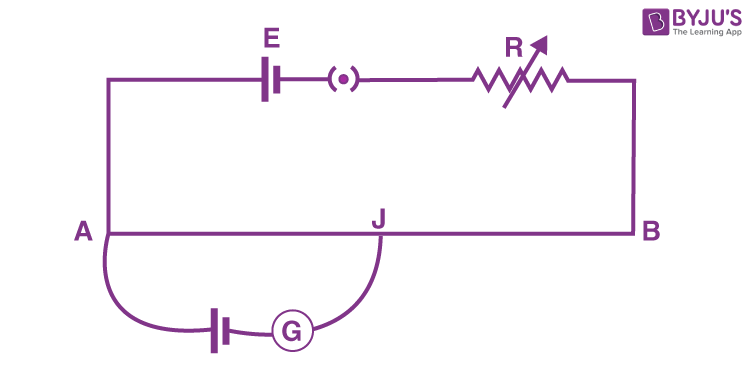Solution:

When R is increased, the electric current in the main circuit will reduce (by V = IR) as the potential is constant. In turn, the potential difference along AB will decrease. Since the R of AB is constant, the potential gradient K = V/AB will decline. In order to balance potential along AB equivalent to the secondary circuit’s potential, the length AJ’ should be bigger than the earlier AJ. Thus, point J changes towards B.

9. While doing an experiment with the potentiometer (Fig 3.5), it was found that the deflection was one-sided, and (i) the deflection decreased while moving from one end A of the wire to the end B: (ii) the deflection increased while the jockey was moved towards end B.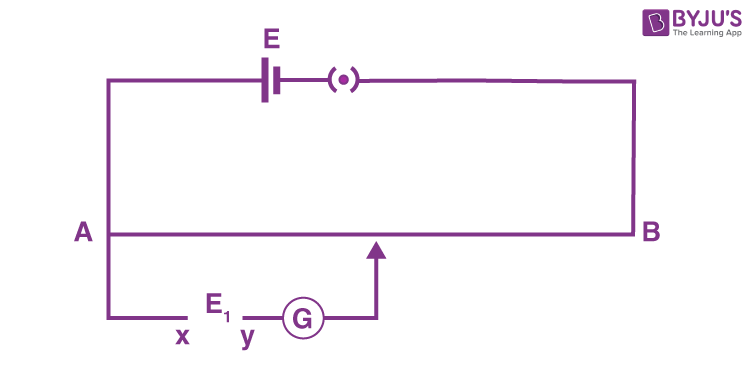• (a) Which terminal of + or -ve of the cell E1, is connected at X in case (i) and how is E1 related to E?
• (a) Which terminal of cell E1 is connected at X in case (ii)?

Solution:

(a) If the electric current in the auxiliary circuit (lower circuit having primary cell) reduces and the potential difference along jockey and A increases. Then deflection in the galvanometer will be one-sided, and the deflection will decrease while displacing from one end A of the wire to the end S. This is only possible when the positive end of the cell E1 is attached to X and E1 > E.

(b) If the electric current in the auxiliary circuit rises, the potential difference between jockey and A rises. Then deflection in the galvanometer is one-sided.

10. A cell of emf E and internal resistance r is connected across an external resistance R. Plot a graph showing the variation of P.D. across R versus R.

Solution:

It is known that

$$\begin{array}{l}I = \frac{ E }{ R + r }\end{array}$$

$$\begin{array}{l}V = IR\end{array}$$

$$\begin{array}{l}\therefore V = \frac{ ER }{ R + r } \,\, ….. \textup{(i)}\end{array}$$

$$\begin{array}{l}V = \frac{ E }{ 1 + \frac{ r }{ R } }\end{array}$$

In this case, r, E are constants.

$$\begin{array}{l}\textup{So, }V \propto \frac{ 1 }{ 1 + \frac{ r }{ R } }\end{array}$$

$$\begin{array}{l}V \propto R\end{array}$$

With the rise in R, the potential difference across R is risen up to the maximum value of E.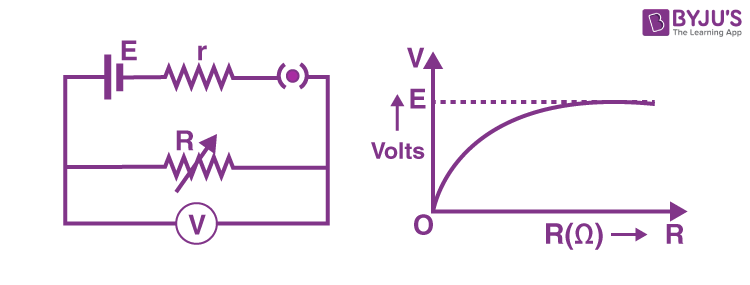11 First, a set of n equal resistors of R each is connected in series to a battery of emf E and internal resistance R. A current I is observed to flow. Then the n resistors are connected in parallel to the same battery. It is observed that the current is increased ten times. What is ‘n’?

Solution:

If n resistance of RO are linked in parallel and series, then

$$\begin{array}{l}R_s = R + R + R \,\, ….. \textup{n times} \end{array}$$

$$\begin{array}{l}\Rightarrow R_p = nR \end{array}$$

$$\begin{array}{l}\frac{1}{R_p} = \frac{1}{R} + \frac{1}{R} + \frac{1}{R} \,\, ….. \textup{ n times} \end{array}$$

$$\begin{array}{l}\Rightarrow \frac{1}{R_p} = \frac{n}{R} \end{array}$$

$$\begin{array}{l}\Rightarrow R_p = \frac{R}{n} \end{array}$$

When n resistors are linked in series and attached to a battery (with emf E), then electric current flows. Therefore,

$$\begin{array}{l}\frac{ E }{ R + nR } = I\end{array}$$

When n resistances are linked in parallel combination, then the electric current in the circuit increases to ten times of I.

$$\begin{array}{l}\therefore \frac{ E }{ R + \frac{ R }{ n } } = 10 I \end{array}$$

$$\begin{array}{l}\frac{ E }{ R + \frac{ R }{ n } } = \frac{ 10 E }{ R + nR }\end{array}$$

$$\begin{array}{l}\frac{ 1 }{ R \left ( 1 + \frac{1}{n} \right ) } = \frac{ 10 }{ R(1 + n) }\end{array}$$

$$\begin{array}{l}10 \left ( 1 + \frac{1}{n} \right ) = 1 + n\end{array}$$

$$\begin{array}{l}10 \left ( 1 + \frac{1}{n} \right ) = 1 + n\end{array}$$

$$\begin{array}{l}10 + \frac{10}{n} – 1 – n = 0\end{array}$$

$$\begin{array}{l}-n + \frac{10}{n} + 9 = 0 ( \textup{multiply both sides by -n} )\end{array}$$

$$\begin{array}{l}n^2 – 10 – 9n = 0 \end{array}$$

$$\begin{array}{l}n^2 – 9n – 10 = 0\end{array}$$

$$\begin{array}{l}n^2 – 10n + 1n – 10 = 0\end{array}$$

$$\begin{array}{l}n(n – 10) + 1(n-10) = 0\end{array}$$

$$\begin{array}{l}\textup{Therefore, }(n+1)(n-10) = 0\end{array}$$

$$\begin{array}{l}n = -1 \textup{ is not possible or } n = 10\end{array}$$

Therefore, there are ten transistors in combination.

12. Let there be n resistors R1 ………. Rn with Rmax = max (R1 …….. Rn) and Rmin = min {R1 ….. Rn}. Show that when they are connected in parallel, the resultant resistance Rp < Rmin and when they are connected in series, the resultant resistance Rs > Rmax. Interpret the result physically.

Solution:

Take Rmax and Rmin are maximum and minimum resistances among all resistance R1 R2 ….. R1. When resistors are linked in parallel, then the equivalent resistance Rp is

$$\begin{array}{l}\frac{ 1 }{ R_v } = \frac{ 1 }{ R_1 } + \frac{ 1 }{ R_2 } + \, …… + \frac{ 1 }{ R }\end{array}$$

Multiplying the two sides by Rmin,

$$\begin{array}{l}\frac{ R_{min} }{ R_p } = \frac{ R_{min} }{ R_1} + \frac{ R_{min} }{ R_2 } + \, …..\, \frac{ R_{min} }{ R_n } \,\,\,\,\, (\textup{among }R_1, R_2, ….., R_n).\end{array}$$

$$\begin{array}{l}\textup{ In this case, in RHS, there exists one term } \frac{ R_{min} }{R_{min} } = 1 \textup{ and the rest of the terms are positive, so we have }\end{array}$$

$$\begin{array}{l}\frac{R_{min}}{R_P} = \frac{R_{min}}{R_1} + \frac{R_{min}}{R_2} + ….. + \frac{R_{min}}{R_{n}} > 1\end{array}$$

This represents the resultant resistance Rp < Rmin.

Therefore, in parallel combination, the resistors’ equivalent resistance Rp is less than any smallest resistance in connection.

If the ‘n’ number of resistance is linked in series, then net resistance is

$$\begin{array}{l}R_s = R_1 + R_2 + R_3 + …… + R_n \,\,\,\,\,\, (b)\end{array}$$

In this case, there is one term possessing resistance Rmax
Thus, in RHS, there should be a term Rmax which possesses maximum value among R1, R2, …….. Rn.

Since every term of equation (b) are positive,

$$\begin{array}{l}R_s = R_1 + R_2 + R_3^+ …….. R_{max} + …… + R_m > R_{max}\end{array}$$

$$\begin{array}{l}R_s > R_{max}\end{array}$$

Thus, in equivalent resistance of the series combination is always more than the maximum resistance (Rmax) among R1, R2, ………. Rn.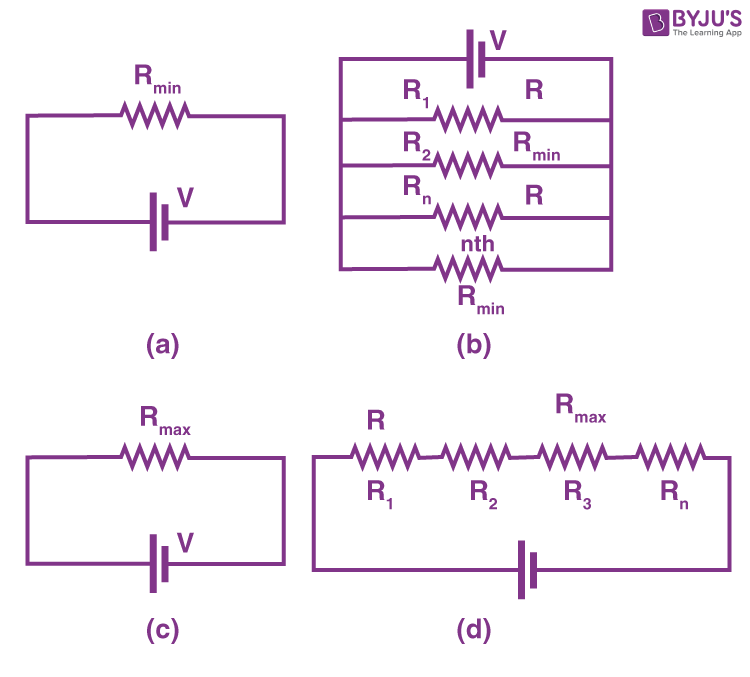13. The circuit in the figure shows two cells connected in opposition to each other. Cell E1 is of emf 6V and internal resistance 2O; cell E2 is of emf 4V and internal resistance 8O. Find the potential difference between points A and B.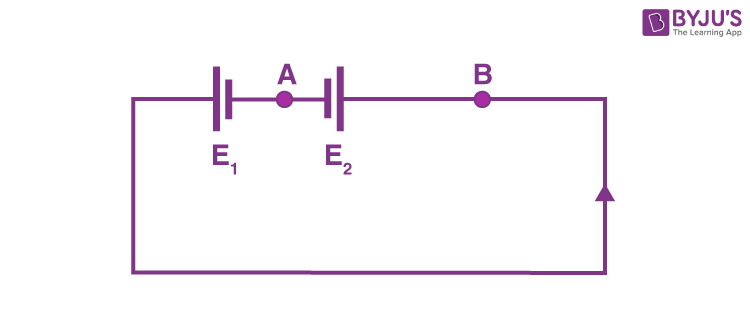Solution:

The current direction in the circuit will be exactly as represented in the diagram. Therefore, point B has a greater potential than A (VB > VA).

$$\begin{array}{l}\textup{Current in the circuit, } I = \frac{ E_1 + E_2 }{r_1 + r_2}\end{array}$$

$$\begin{array}{l}= \frac{ (6 – 4) }V { (2 + 8)\Omega } = 0.2 \textup{amp}\end{array}$$

The potential across E1 and E2 are

$$\begin{array}{l}E_1 = V – Ir_1 = 6 – 0.2 \times 2 = 6 – 0.4 = 5.6 V\end{array}$$

$$\begin{array}{l}E_2 = V + Ir_2 = 4 + 0.2 \times 8 = 4 + 1.6 = 5.6V\end{array}$$

Therefore, the potential between B and A is

$$\begin{array}{l}E_2 = 5.6 Volt\end{array}$$

Since the current is flowing from B to A, the potential at point B is greater than A.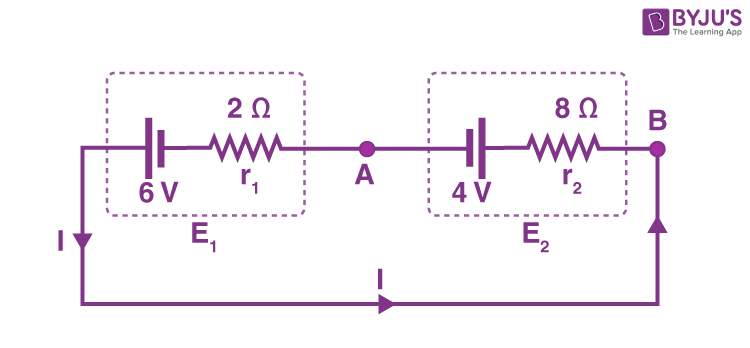14. Two cells of the same emf E but internal resistance r1 and r2 are connected in series to an external resistor R. What should be the value of R so that the potential difference across the terminals of the first cell becomes zero?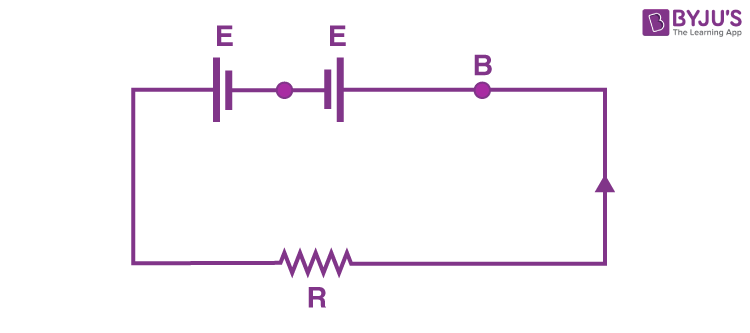Solution:

The current flowing through the circuit is

$$\begin{array}{l}I = \frac{ E_{eq} }{ R + r_1 + r_2} \, …………… (i) \, (\textup{ In series, } E + E = 2E)\end{array}$$

Then, the potential drop along the first cell is

$$\begin{array}{l}V_1 = E – Ir_1\end{array}$$

As per the given scenario

$$\begin{array}{l}V_1 = 0\end{array}$$

Thus,

$$\begin{array}{l}E – Ir_1 = 0\end{array}$$

$$\begin{array}{l}E- \left ( \frac{ 2E }{ r_1 + r_2 + R } \right )r_1 = 0 \, ……. \textup{from } (i)\end{array}$$

$$\begin{array}{l}\frac{ 2r_1E }{ r_1 + r_2 + R } = E\end{array}$$

$$\begin{array}{l}\Rightarrow r_1 + r_2 + R = 2r_1 \end{array}$$

$$\begin{array}{l}R = r_1 – r_2\end{array}$$

15. Two conductors are made of the same material and have the same length. Conductor A is a solid wire of diameter 1mm. Conductor B is a hollow tube with an outer diameter of 2mm and an inner diameter of 1mm. Find the ratio of resistance RA to RB.

Solution:

We know that

$$\begin{array}{l}R = \frac{ \rho l }{A}\end{array}$$

Let us assume that the conductor is made up of a substance of resistivity ‘p (rho)’ and the conductor’s length be ‘I’. The diameter of the wire A, d = 1mm

$$\begin{array}{l}\textup{Radius of the wire, } r = \frac{d}{2} = 0.5 \times 10^{-3}m\end{array}$$

$$\begin{array}{l}\textup{ Resistance of the Wire A, } R_A = \frac{ \rho l }{ \pi (0.5 \times 10^{-3})^2 }\end{array}$$

Now let’s find the resistance of wire B.

$$\begin{array}{l}R = \frac{ \rho l }{ A }\end{array}$$

Let us assume that the conductor is composed of a substance of resistivity ‘p (rho)’ and the conductor’s length be ‘I’. The diameter of the wire B, d1 = 1mm

The inner radius of the wire is

$$\begin{array}{l}r_1 = \frac{ d_1 }{ 2} = 0.5 \times 10^{-3}\end{array}$$

The outer diameter of the wire B, d2 = 2mm

$$\begin{array}{l}\textup{ Outer radius of the wire, } r_2 = \frac{ d_2 }{ 2 } = 1 \times 10^{-3}m\end{array}$$

The resistance of the wire B,

$$\begin{array}{l}R_B = \frac{ \rho l }{ \pi ( (r_2)^2 – (r_1)^2 ) }\end{array}$$

$$\begin{array}{l}= \frac{ \rho 1 }{ \pi ( ( 1 \times 10^{-3} )^2 – (0.5 \times 10^{-3})^2 ) }\end{array}$$

The ration of RA and RB is

$$\begin{array}{l}\frac{ R_A }{ R_B } = \frac{ (1 \times 10^{-3} )^2 – (0.5 \times 10^{-3})^2 }{ (0.5 \times 10^{-3})^2 }\end{array}$$

$$\begin{array}{l}\frac{ R_A }{ R_B } = \frac{ 3 }{ 1 }\end{array}$$

The ratio of resistance RA to RB is 3:1.

16. Suppose there is a circuit consisting of only resistances and batteries. Suppose one is to double (or increase it to n-times) all voltages and all resistances. Show that currents are unaltered. Do this for the circuit shown here.Solution:

Scenario A: Let us assume a circuit composed of external resistance R1, R2 ………, linked with some batteries E1, E2, E3 ……. possessing their internal resistances r1, r2, r3 ………..

Take the emf, internal resistance, and equivalent resistance of the given combination as Req, Eeq, and req, respectively. Therefore, the current passing in the circuit is

$$\begin{array}{l}I_1 = \frac{ nE_{eq}}{ R_{eq} + r_{eq} }\end{array}$$

Then the cells and resistance are again linked in a way that their resultant resistance, emf, and internal resistance are nReq, nEeq, and nreq and respectively. Therefore, again electric current in the new circuit is

$$\begin{array}{l}I_2 = \frac{ nE_{eq} }{ nR_{eq} + nr_{eq} }\end{array}$$

$$\begin{array}{l}I_2 = \frac{ nE_{eq} }{ n[ R_{eq} + r_{eq} ] }\end{array}$$

$$\begin{array}{l}= \frac{ E_{\theta q} }{ R_{ \theta q} + r_{eq} } = I_1\end{array}$$

Thus, the electric current stays the same if the R, E, and r of a circuit are raised by n times, i.e. nR, nE, nr.

1. Two cells of voltage 10V and 2V and internal resistances 10O and 5O, respectively, are connected in parallel with the positive end of the 10V battery connected to the negative pole of the 2V battery (Fig 3.8). Find the effective voltage and effective resistance of the combination.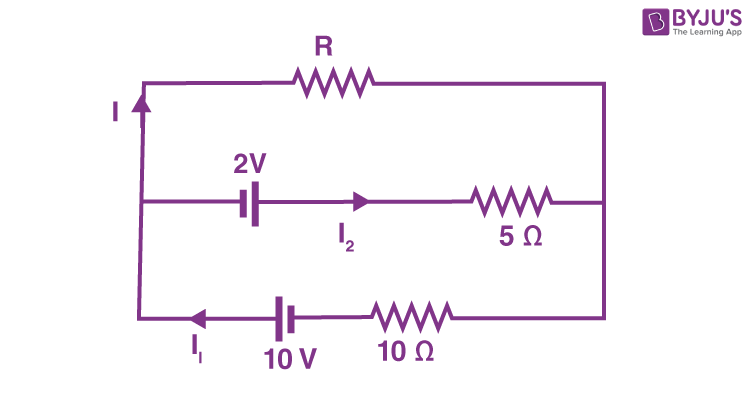Solution:

According to the junction rule, at A

$$\begin{array}{l}I_1 = I + I_2\end{array}$$

When Kirchhoffs rule on loop ADEF and loop BCEF

$$\begin{array}{l}10 = IR + 10I_1\end{array}$$

$$\begin{array}{l}2 = 5I_2 – IR\end{array}$$

$$\begin{array}{l}\therefore 2 = 5(I_1 – I ) – IR \end{array}$$

$$\begin{array}{l}\textup{ From the above equations, we get } 4 = 10 I_{1} – 10I – 2IR\end{array}$$

Subtract the above equation from the first equation,

$$\begin{array}{l}6 = 3 I R + 10 I\end{array}$$

$$\begin{array}{l}3 IR + 10I = 6\end{array}$$

$$\begin{array}{l}I(3R + 10) = 2 \times 3\end{array}$$

$$\begin{array}{l}\frac{ I (3R +10) }{ 3 } = 2\end{array}$$

$$\begin{array}{l}2 = I \left ( R + \frac{10}{3} \right )\end{array}$$

The resultant potential difference due to the two batteries is Veq. It is will be along resistance R.

$$\begin{array}{l}\therefore V_{eq} = I (R + R_{eq}) \end{array}$$

Req is the circuit’s resistance except for R.

$$\begin{array}{l}V_{eq} = 2 \textup{ Volts and } R_{eq} = \frac{10}{3} \Omega \end{array}$$

2. A room has AC run for 5 hours a day at a voltage of 220V. The wiring of the room consists of Cu of 1 mm radius and a length of 10m, and power consumption per day is ten commercial units. What fraction of it goes in the joule heating in wires? What would happen if the wiring is made of the same dimensions?

$$\begin{array}{l}[ \rho _{cu} = 1.7 \times 10^{-8} \Omega m, \,\, \rho _{Al} = 2.7 \times 10^{-8} \Omega m] \end{array}$$

Solution:

The entire energy used in five hours a day by AC and wiring is 10kWh

Therefore, the energy used in one hour by AC and wiring is 2kWh.

The total power of the AC and wire is 2000W.

$$\begin{array}{l}P = VI\end{array}$$

$$\begin{array}{l}I = \frac{P}{V} \end{array}$$

$$\begin{array}{l}= \frac{2000}{220} \cong 9.0A\end{array}$$

Take P0 as the power of wiring then,

$$\begin{array}{l}P_{0} = I^2 R_{W} \, [R_W = \textup{resistance of wiring}]\end{array}$$

$$\begin{array}{l}= 9 \times 9\cdot \frac{P!}{A}\end{array}$$

$$\begin{array}{l}= \frac{ 9 \times 9 \times 1.7 \times 10^{-8} \times 10 }{ 3.14 \times 1 \times 10^{-3} \times 1 \times 10^{-3} }\end{array}$$

$$\begin{array}{l}= \frac{ 81 \times 17 \times 10^{-8 + 6} }{ 3.14 }\end{array}$$

$$\begin{array}{l}= \frac{ 1377 }{ 3.14 } \times 10^{-2}\end{array}$$

$$\begin{array}{l}= 4.38 = 4.4 Watt\end{array}$$

Thus, the energy loss in the wiring

$$\begin{array}{l}\cong 4.4 J/sec \end{array}$$

The fractional loss due to wire heating

$$\begin{array}{l}= \frac{ 4.4 }{ 2000 } \times 100 \% \end{array}$$

$$\begin{array}{l}= 0.22 \% \end{array}$$

$$\begin{array}{l}\frac{ P_A \,(wiring) }{ P_{Cu} \, (wiring) } = \frac{ I^2R_A }{I^2R_{Cu}}\end{array}$$

$$\begin{array}{l}= \frac{ \rho Ai \frac{I_A}{A_{Ai}} }{ \rho Cu \frac{I_{Cu}}{ A_{Cu} }} \textup{ as } 1_{AI} = L_{Cu} \textup{ and } A_{AI} = A_{Cu} \end{array}$$

$$\begin{array}{l}\frac{ P_{A} }{ P_{Cu}} = \frac{ \rho _{A} }{ \rho _{Cu} }\end{array}$$

$$\begin{array}{l}P_{A} = \frac{ 2.7 \times 10^{-8} }{ 1.7 \times 10^{-8} } \times 4.4 Watt\end{array}$$

Therefore, the loss of power in Al wiring is 7 Watts.

3. In an experiment with a potentiometer, VB = 10V. R is adjusted to be 50O (Fig. 3.9). A student wanting to measure voltage E1 of a battery (approx 8V) finds no null point possible. He then diminishes R to 10O and is able to locate the null point on the last (4th) segment of the potentiometer. Find the resistance of the potentiometer wire and potential drop per unit length across the wire in the second case.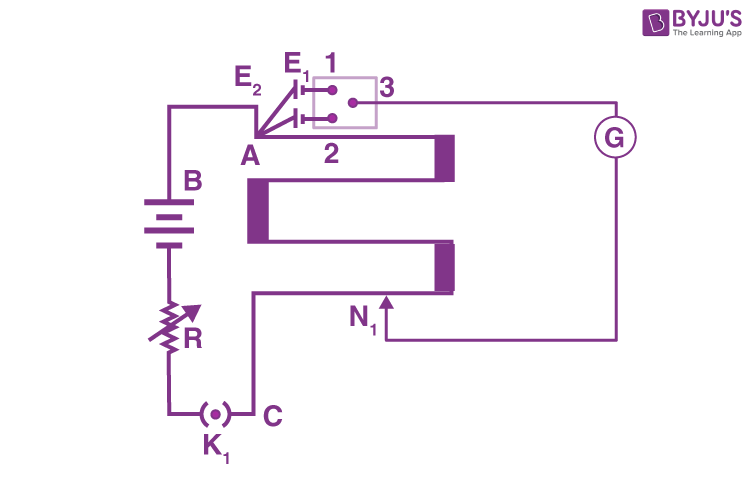Solution:

Consider R as the potentiometer wire’s resistance.

$$\begin{array}{l}\textup{Variable resistance, R} = 50\Omega \end{array}$$

I is the electrical current in the primary, which is located at EB = 10V.

$$\begin{array}{l}I \frac{ V_B }{ R + R’ } \Rightarrow \frac{10}{ 50 + R } = I ( \textup{ in primary circuit})\end{array}$$

Potential difference along the potentiometer wire is

$$\begin{array}{l}V’ = IR'\end{array}$$

$$\begin{array}{l}\textup{ From } I \, V’ = \frac{10R’}{50 + R}\end{array}$$

Since R = 50O, the null point cannot be acquired by 8 Volt. Therefore, V < 8 Volt.

$$\begin{array}{l}\frac{ 10 R’ }{ 50 + R’}\,\, (\textup{no balance point})\end{array}$$

As 50 + R’ is positive, therefore, we can multiply the above relation by a positive number, and we get

10R’ < 400 + 8R’

2R’ < 400

R’ < 200

The null point acquired by R = 10O.

V’ > 8 at balance point. Thus, it is possible when

$$\begin{array}{l}\frac{ 10R’ }{ 10 + R } > 8 \, ( \textup{as from I, R = 10})\end{array}$$

Multiply the above equation by the positive value 10 + R’ to both sides

10R’ > 80 + 8R

2R’ > 80

R’ > 40

Since the null point is acquired on the fourth segment or at 3\4 of the total length. Thus, at (3\4)R’ (no balance point)

$$\begin{array}{l}\textup{Or } \frac{ 10 \times \frac{3}{4}R’ }{ 10 + R } < 8 \end{array}$$

$$\begin{array}{l}\therefore 7.5R’ < 80 + 8R \end{array}$$

$$\begin{array}{l}-0.5R’ < 80\end{array}$$

$$\begin{array}{l}- R’ < 160 \end{array}$$

$$\begin{array}{l}R’ > -160\end{array}$$

R’ can never be a negative value. Therefore -160O is considered as 160O

$$\begin{array}{l}\textup{So, }[160 < R’ < 200] …. V\end{array}$$

Any of the R’s between 200O and 160O will attaina null point. As the null point is on the last fourth segment of the potentiometer wire, the potential drop along 400cm wire > 7 Volt.

Therefore, K(400cm) > 8V (at balance point)

$$\begin{array}{l}K > \frac{8}{400}Volt/cm \end{array}$$

$$\begin{array}{l}K > \frac{8}{4}Volt/,\end{array}$$

$$\begin{array}{l}K > 2Volt/m\end{array}$$

Since the balance point is at the fourth wire, therefore no balance point at 3m.

$$\begin{array}{l}K (3) < 8 (\textup{no balance point})\end{array}$$

$$\begin{array}{l}K < \frac{ 8 }{ 3 } Volt/m\end{array}$$

$$\begin{array}{l}K < 2 \frac{2}{3}Volt/m\end{array}$$

$$\begin{array}{l}\therefore \left [ 2 \frac{2}{3}V/m \right > K > 2Volt/m ] \end{array}$$

$$\begin{array}{l}\therefore \left [ 2 \frac{2}{3} V/m > K > 2Volt/m \right ] \end{array}$$

4. (a) Consider the circuit in the Figure. How much energy is absorbed by electrons from the initial state of no current (ignore thermal motion) to the state of drift velocity?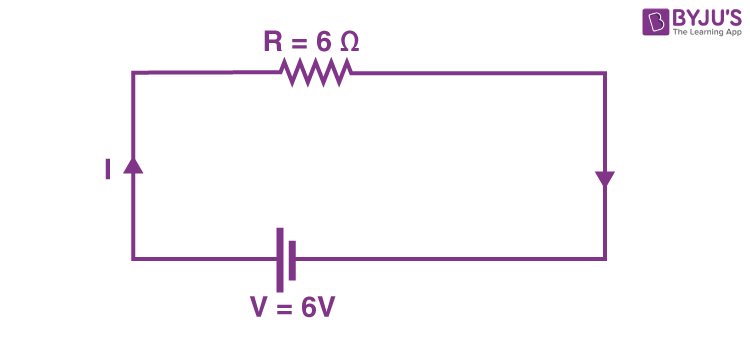(b) Electrons give up energy at the rate of RI2 per second to the internal energy. What time scale would one associate with energy in problem (a)? N = no of electrons/volume = 1029/m3, length of circuit = 10cm, cross-section = A = (1mm)2.

Solution:

(a) By using ohm’s law, current I is represented by

$$\begin{array}{l}I = \frac{6V}{6\Omega } = 1A\end{array}$$

$$\begin{array}{l}i = neAv_d\end{array}$$

$$\begin{array}{l}v_d = \frac{i}{neA}\end{array}$$

On substituting the quantities,

$$\begin{array}{l}n \textup{ = the number of electrons/volume } = 10^{29}/m^3\end{array}$$

Circuit length = 10cm

Cross section area A = 1(mm)2

$$\begin{array}{l}v_d = \frac{ 1 }{ 10^{29} \times 1.6 \times 10^{-19} \times 10^{-6} }\end{array}$$

$$\begin{array}{l}= \frac{1}{1.6} \times 10^{-4} m/s\end{array}$$

Thus, the energy absorbed in the form of kinetic energy is represented by

$$\begin{array}{l}KE = \frac{1}{2} m_ev^2_d \times nAI\end{array}$$

$$\begin{array}{l}= \frac{1}{2} \times 9.1 \times 10^{31} \times \frac{1}{2.56} \times 10^{20} \times 10^{8} \times 10^6 \times 10^1\end{array}$$

$$\begin{array}{l}= 2 \times 10^{-17} J\end{array}$$

(b) Loss of power is represented by

$$\begin{array}{l}P = I^2R = 6 \times 1^2 = 6W = 6J/s\end{array}$$

$$\begin{array}{l}P = \frac{E}{t}\end{array}$$

$$\begin{array}{l}\therefore E = P \times t \end{array}$$

$$\begin{array}{l}\textup{or } t = \frac{E}{P}\end{array}$$

$$\begin{array}{l}= \frac{2 \times 10^{-17} }{ 6 } = 10^{-17}s\end{array}$$

#### Current Electricity Class 12 Physics One Shot & Mind Maps (Chapter 3)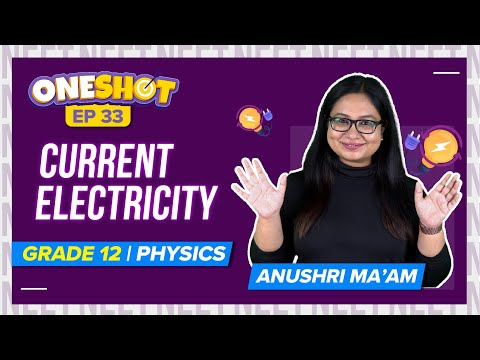#### Current Electricity Class 12 Physics One Shot (Full Chapter Revision)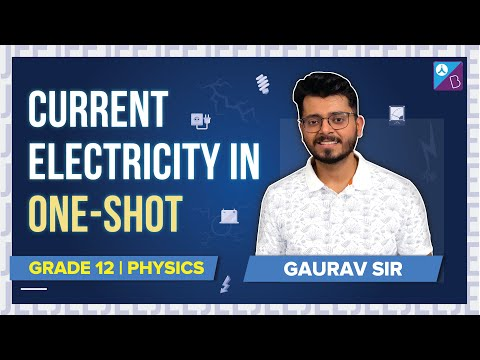s

#### Current Electricity Class 12 Physics One Shot#### Current Electricity Class 12 Physics Crash Course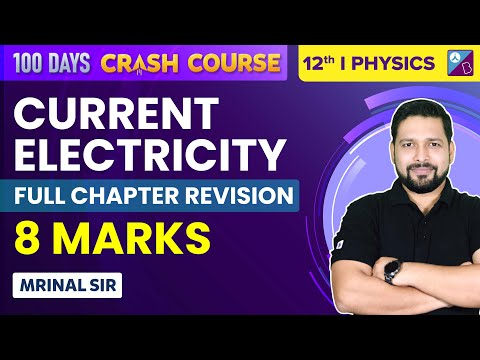s